# Square Root

The square root of a number is a value that, when multiplied by itself, gives the number. The SQRT function in Excel returns the square root of a number.

1. First, to square a number, multiply the number by itself. For example, 4 * 4 = 16 or 4^2 = 16.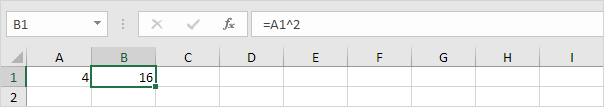Note: to insert a caret ^ symbol, press SHIFT + 6.

2. The square root of 16 is 4.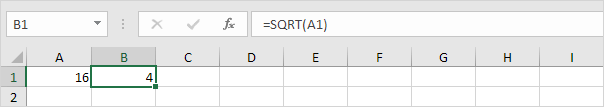3. Instead of using the SQRT function, you could also use an exponent of 1/2. Don't forget the parentheses.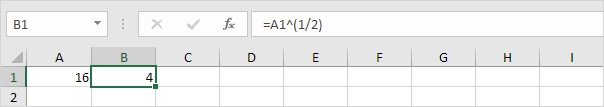4. If a number is negative, the SQRT function returns the #NUM! error.5. You can use the ABS function to remove the minus sign (-) from a negative number.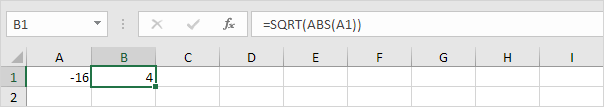Excel has no built-in function to calculate the nth root of a number. To calculate the nth root of a number, simply raise that number to the power of 1/n.

6. For example, 5 * 5 * 5 or 5^3 is 5 raised to the third power.7. The cube root of 125 is 5.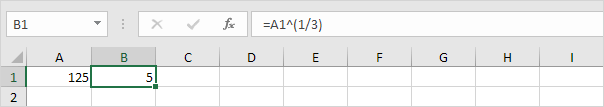8. For example, 2 * 2 * 2 * 2 * 2 * 2 or 2^6 is 2 raised to the sixth power.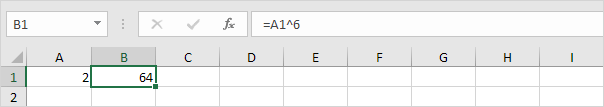9. The sixth root of 64 is 2.Go to Next Chapter: Ribbon